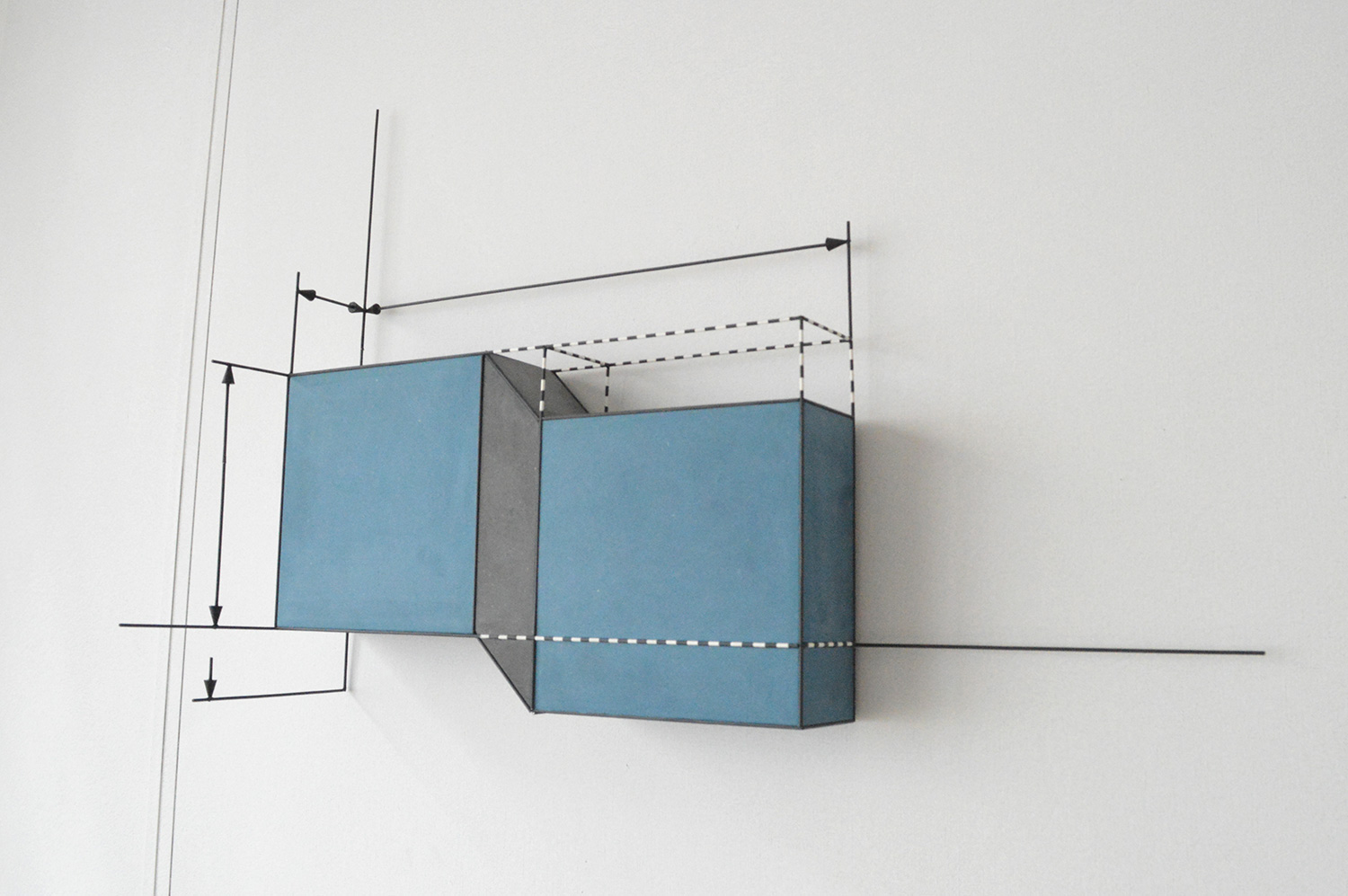rick van meel | work | news | about Epoxy Joint | 2018 | mdf, steel, plastic | 65 x 24 x 46 cm | SOLD"Epoxy Joint" A joint between two slabs A and B is filled with a flexible epoxy that bonds securely to the concrete (see figure). The height of the joint is b = 100mm, its length perpendicular to the plane of the paper is L = 1 m, and its thickness is t = 12 mm. Under the action of shear forces V, the slabs displace vertically through the distance d = 0.05 mm relative to each other. (a) What is the average shear strain γ in the epoxy? (b) What is the magnitude of the forces V if G = 965 MPa for the epoxy? * Gere, J.M. en Timoshenko, S. P. (1991) Mechanics of materials (3e SI ed.) 1. A point is that which has no part. 2. A line is breadthless length. 3. The ends of a line are points. 4. A straight line is a line which lies evenly with the points on itself. 5. A surface is that which has length and breadth only. 6. The edges of a surface are lines. 7. A plane surface is a surface which lies evenly with the straight lines on itself. 8. A plane angle is the inclination to one another of two lines in a plane which meet one another and do not lie in a straight line. 9. And when the lines containing the angle are straight, the angle is called rectilinear. 10. When a straight line standing on a straight line makes the adjacent angles equal to one another, each of the equal angles is right, and the straight line standing on the other is called a perpendicular to that on which it stands. 11. An obtuse angle is an angle greater than a right angle. 12. An acute angle is an angle less than a right angle. 13. A boundary is that which is an extremity of anything. 14. A figure is that which is contained by any boundary or boundaries. Et cetera.* Byrne, O. (2013) The Elements of Euclid (1e ed.) ^